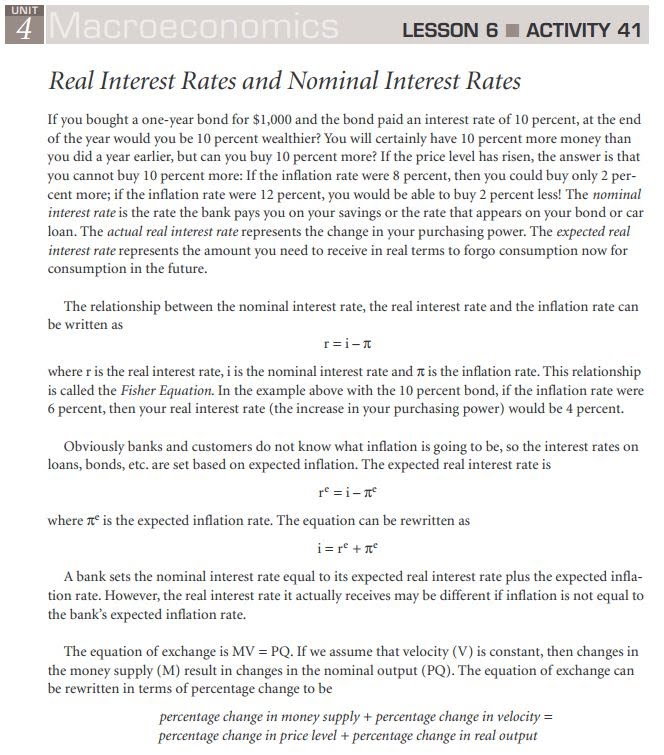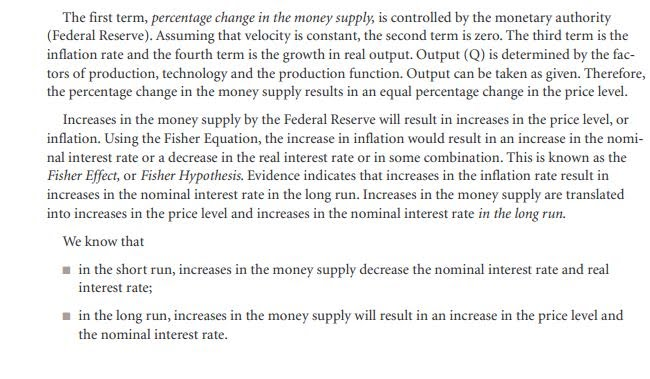A41-Real & Nominal Interest Rates
First name *
Last name *
SCHOOL email address--TYPE IT CORRECTLY for CREDIT *
Class period *Compute the actual real interest rates for 1989 through 20011.
Nominal interest rate - inflation = Real interest rate
1990-Nominal 7.89% Inflation 5.39% *
Real interest rate?
1992-Nominal 3.89 Inflation 3.03% *
Real interest rate?
1995-Nominal 5.94% Inflation 2.81% *
Real interest rate?
1997-Nominal 5.63% Inflation 2.34% *
Real interest rate?
1999-Nominal 5.08% Inflation 2.19% *
Real interest rate?
2000-Nominal 6.11% Inflation 3.38% *
Real interest rate?
2001-Nominal 3.49% Inflation 2.83% *
Real interest rate?
2003-Nominal 1.24% Inflation 2.27 *
Real interest rate?
2004-Nominal 1.89% Inflation 2.68% *
Real interest rate?
2006-Nominal 4.94% Inflation 3.24% *
Real interest rate?
2007-Nominal 4.53% Inflation 2.85% *
Real interest rate?
2008-Nominal 1.83% Inflation 3.85% *
Real interest rate?
2009-Nominal 0.47% Inflation -0.34% *
Real interest rate?
2010-Nominal 0.32% Inflation 1.64% *
Real interest rate?
2012-Nominal 0.17% Inflation 1.7% *
Real interest rate?
2013-Nominal 0.09% Inflation 1.5% *
Real interest rate?
Refer to the graph below for nominal and real interest rates.
2. Has the actual real interest rate stayed constant? *
3. If it has not, explain why you think the real rate has not been constant. *
4. For what years has the actual real interest rate remained nearly constant? *
Type in separated by a comma
5. Frequently, economists argue that the monetary authorities should try to maintain a steady real interest rate. Explain why you think a steady real rate of interest is important to the economy *
6. How does the current real interest rates (2007-2012) compare to the real interest rates of the 1990's *
PART B. Suppose that initially the economy is at the intersection of AD & SRAS on the LRAS curve (in long run equilibrium). The Fed decides to implement monetary policy to increase the level of employment PAST the full employment level-inflationary gap..
7. What happens to each of the following in the short run? *
Increase
Decrease
Unchanged
Real GDP (output)
Price Level (inflation)
Unemployment
Nominal wages
Nominal interest rates
Real interest rates
8. What happens to each of the following in the LONG RUN? *
LONG RUN
Increase
Decrease
Unchanged
Real GDP (output)
Price Level (inflation)
Unemployment
Nominal wages
Nominal interest rates
Real interest rates
9. Explain the difference between the long run and short run regarding interest rates and the economy *